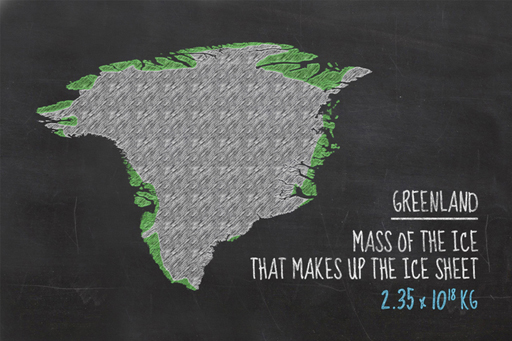Basic science: understanding numbers

Start this free course now. Just create an account and sign in. Enrol and complete the course for a free statement of participation or digital badge if available.

Free course

# 2.4.1 Density of the Greenland ice sheetFigure 8 What would happen if this much ice were to melt?

Since density is the amount of mass in a given volume, it follows that the mass of an object can be calculated if you know the density and the volume. We have already estimated the volume of the Greenland ice sheet previously. It turned out to be 2,565,000 km3. The density of ice is 917 kg/m3, so you can now calculate the mass of ice.

If you start with just one cubic kilometre: 1 km3 contains 1,000,000,000 (or 1 × 109, or a billion) cubic metres, each with a mass of 917 kilograms, so 1 km3 weighs 917,000,000,000 kg. This can also be written, you remember, as 9.17 × 1011 kg. This is the mass of each cubic kilometre of ice.

But there are 2,565,000 cubic kilometres of ice in the ice sheet, so you must multiply your answer by this number to get the total. 2,565,000 × 917,000,000,000 gives us a value of 2,352,105,000,000,000,000, or in scientific notation, 2.35 × 1018 kg of ice.